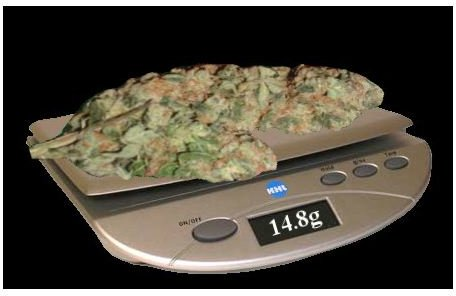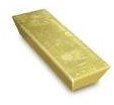# Tricks of Unit Conversion: How to Convert Ounces to Grams, Both Troy & Avoirdupois Ounce

Unit conversion is a very popular topic of many physics and math problems. Of course, as we are living in a world that has various dimensioning systems (imperial and metric are two popular examples), it is mandatory to be aware of the conversion factors. Knowing how to convert ounces to grams, kilograms to pounds, kilometers to miles and other units can easily become a routine task when, for example, you travel abroad.

## A Coarse ApproximationSo, how to convert ounces to grams? A very rough approximation is “multiply by thirty”.

One ounce is about 30 grams, so when someone is talking about 5 ounces it is approximately 150 grams, making a kilogram (1000 gram) roughly equal to 33.3 ounces.

However, if we are talking about kilograms, we should use a more fine approximation of 28 grams per ounce. This gives roughly 35 ounces in a kilogram. Of course, those are very rough approximations that are useful to get a general idea of a weight of certain product. To solve math problems, you will need more precise numbers.

## By Definition

As the ounce is 1/16 of a pound and gram is 1/1000 of a kilogram, the definition of conversion used in the UK since 1963 and in the USA since 1959 says that, “”one pound shall be 0.45359237 kilogram exactly.” This defines the ounce/gram conversion as well.

By this definition, we get 1 ounce = 28.349523125 grams. As usual, for most practical purposes, using second decimal digit is acceptable accuracy. For this reason, we should mostly use 1ounce = 28.35g.

## An Example of How to Convert Ounces to Grams

Sample Question:

If the price of an ounce of gold is \$1417.50, how much should a person pay for half a kilogram of gold?

Solution:

First of all, let’s get a price per gram. As we said, ounce is 28.35g. Thus, the cost is \$1417.5 per 28.35 gram. Per ounce it is \$1417.5/28.35 = \$50 per gram. For half a kilo (500 gram) it will be 50*500 = \$25,000 dollars. Excellent!

Note:

Unfortunately, this is not entirely correct, and not because of approximation. If we had used the exact 1ounce = 28.349523125 ration, we would get \$25,000 dollars and 42 cents, which is really not significant.

The thing is, for precious metals they use “another” ounce, called the “troy ounce”

## Troy OunceThis unit, used only for weights of precious metals (Gold, Platinum, Silver etc.), is defined to be slightly “heavier” than the “regular” (avoirdupois) ounce.

Equal to exactly 480 grains (opposed to 437.5 grains – 7000 grains per pound/16 – for the avoirdupois ounce), it is equal to 31.1034768 grams.

## Resolving the Question

Now, let’s get the correct solution with the updated numbers. Do you remember how to convert ounces to grams? Just don’t forget to use a troy ounce this time:

\$1417.5/ounce = \$1417.5/31.1034768 = \$45.5736833/g

500 gram will then cost \$22786.84

## References and Image Credits

https://www.goldprice.org/gold-price.html

https://www.asknumbers.com/OuncesToGramsConversion.aspx

Image Credits:

https://www.goldprice.org/gold-prices/1003-Gold-Coins/1005-GoldMoney.htm

https://usspost.com/grams-to-ounces-19778/grams-to-ounces